# Control of reaction-diffusion under state constraints

Author: - 30 March 2020

Reaction-diffusion equations appear frequently in natural phenomena such as:

• Population dynamics and invasion of species (see ).
• Neuroscience, where models for neuronal impulses exhibit traveling waves (see ).
• Chemical Reactions: modeling the evolution of concentrations of chemicals (see ).
• In evolutionary game theory (see [4, 5]).
• Magnetic systems in material science and their phase transitions (see ).
• Linguistics, we refer to  where the authors consider reaction-diffusion for analyzing language shift by means of a traveling wave.

In the majority of the systems mentioned above, the state $u$ of an equation of the type

represents a population, concentration or proportion. For this reason, any model intending to predict the behavior of such quantity must fulfill a maximum principle .

In this group of tutorials, our aim is to explain the phenomenology arising when considering a control problem in the contexts mentioned above.

In general, the controllability of parabolic equations has been widely studied (for instance [9,10,11]). However, in this literature, the requirement that the trajectory has to be positive or between prescribed bounds was not a concern.

In Figure 1 one can see a boundary control of the semilinear equation

with $a$ being the control and the target function $v\equiv 0.33$.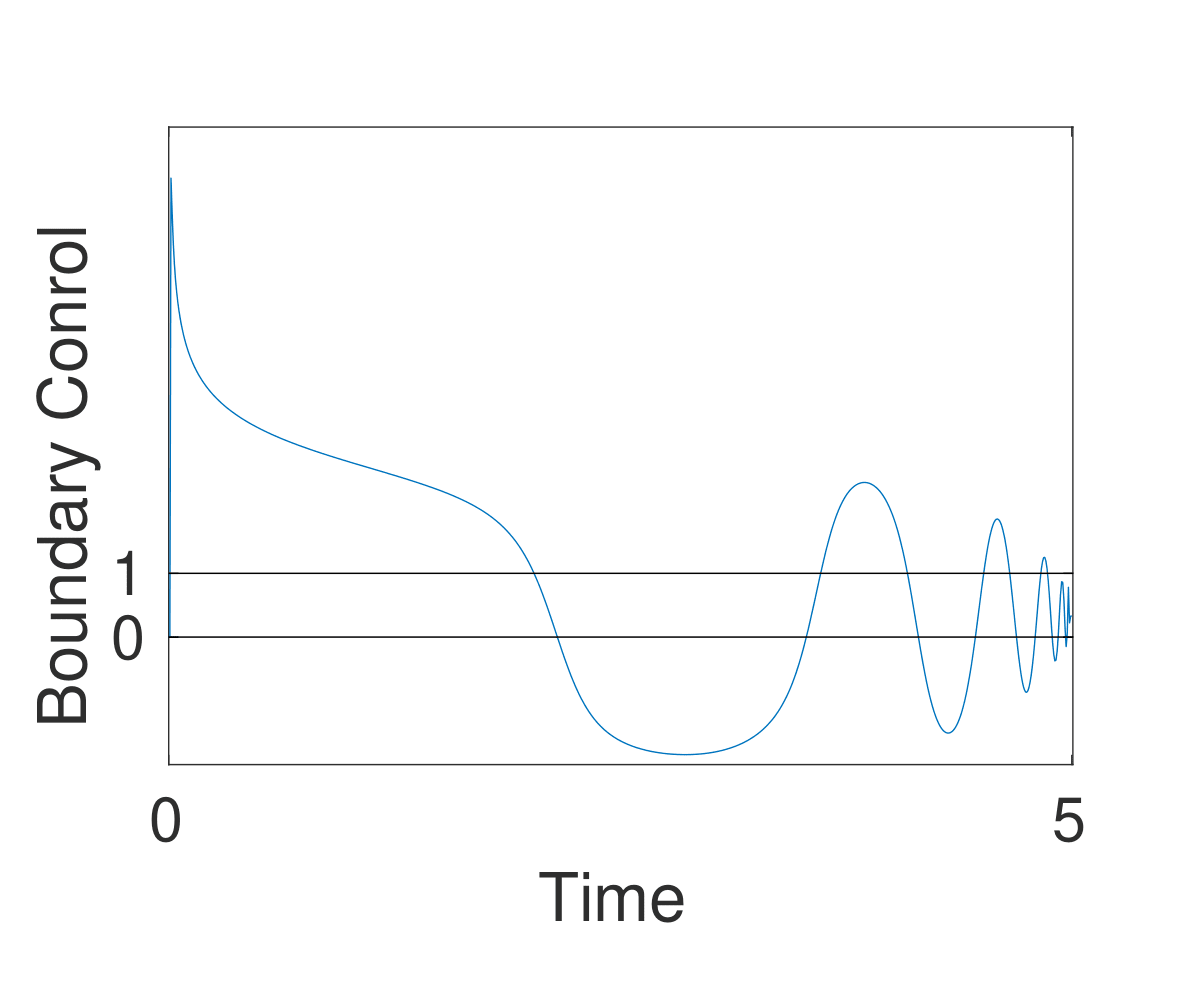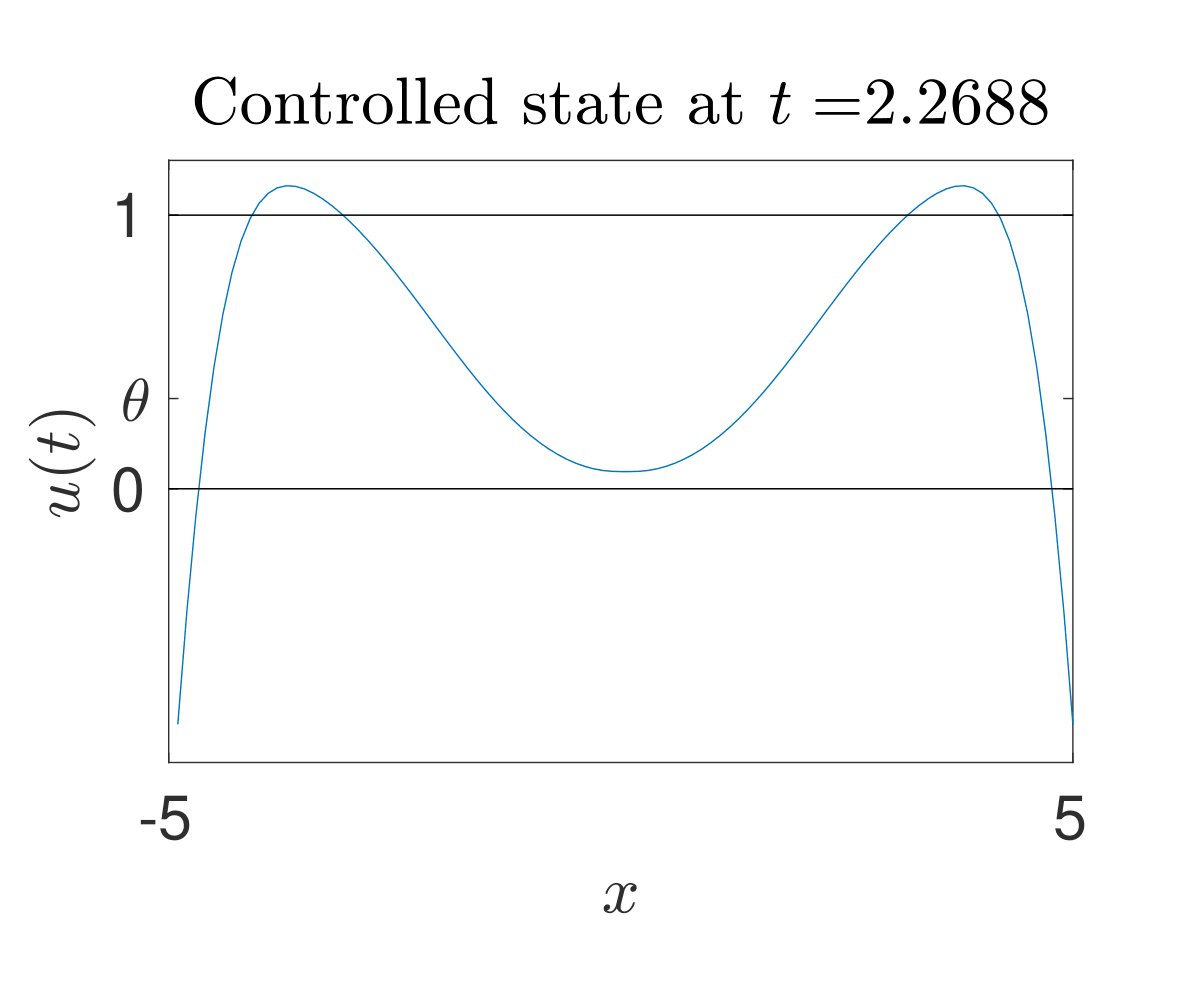Control from $u(0)\equiv 0$ to $u(T)\equiv 0.33$.

This model models the evolution of a proportion, and we observe that the control does not preserve the positivity of the state neither the meaningful upper bound.

From the application point of view of several of the applications mentioned, any control action proposed must fulfill that the associated trajectory has meaning.

The ideas exposed in this tutorial group are the following:

• The presence of state-constraints can create intrinsic obstructions for achieving the controllability. This is due to the emergence on non-trivial solutions and the comparison principle. The topic is treated in this blog.
•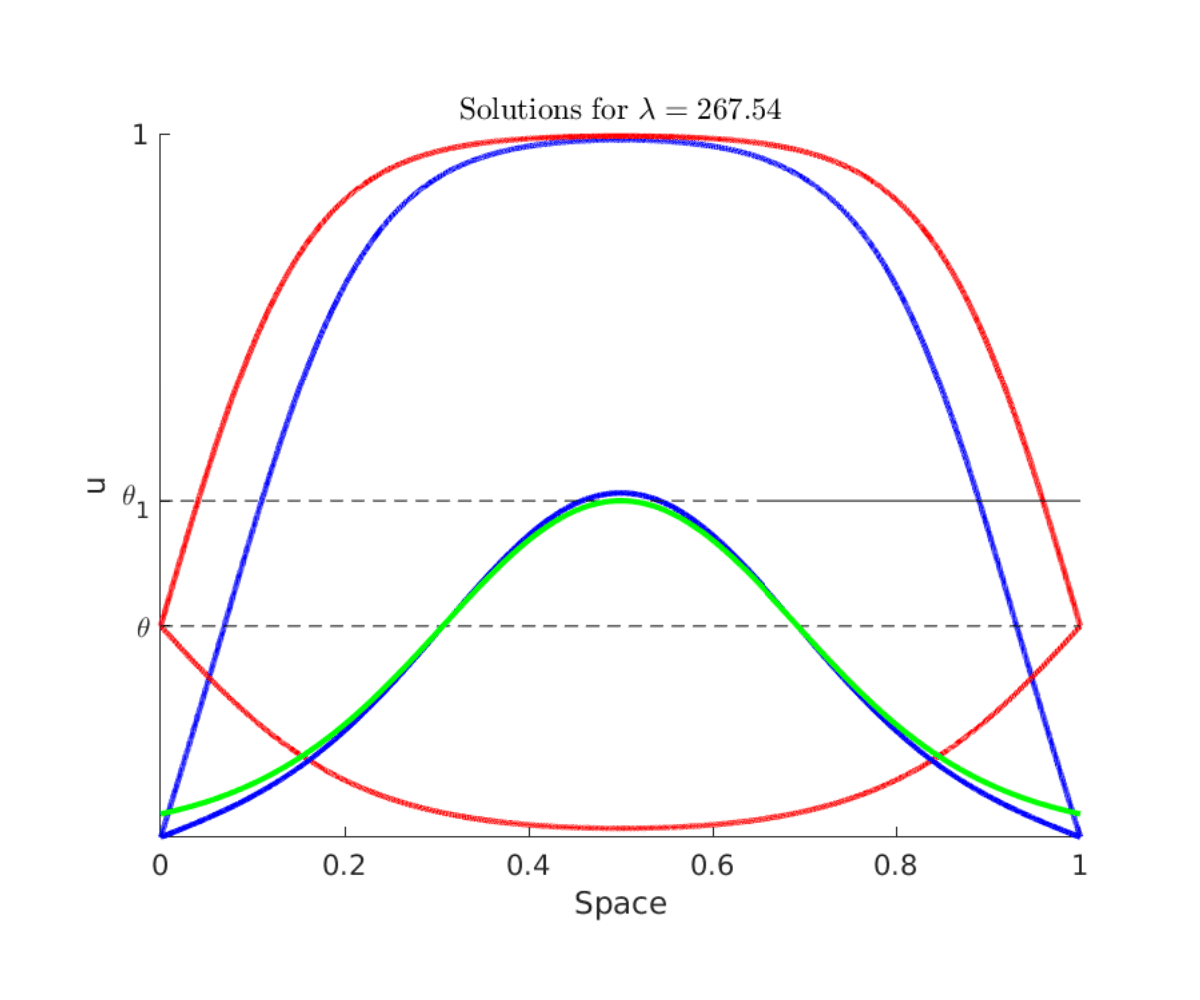• In order to prove the existence of controls one can use the stair-case method. This method relies on the construction of paths of steady-states that the controlled trajectory can follow. One can find more information about these constructions in this blog.
•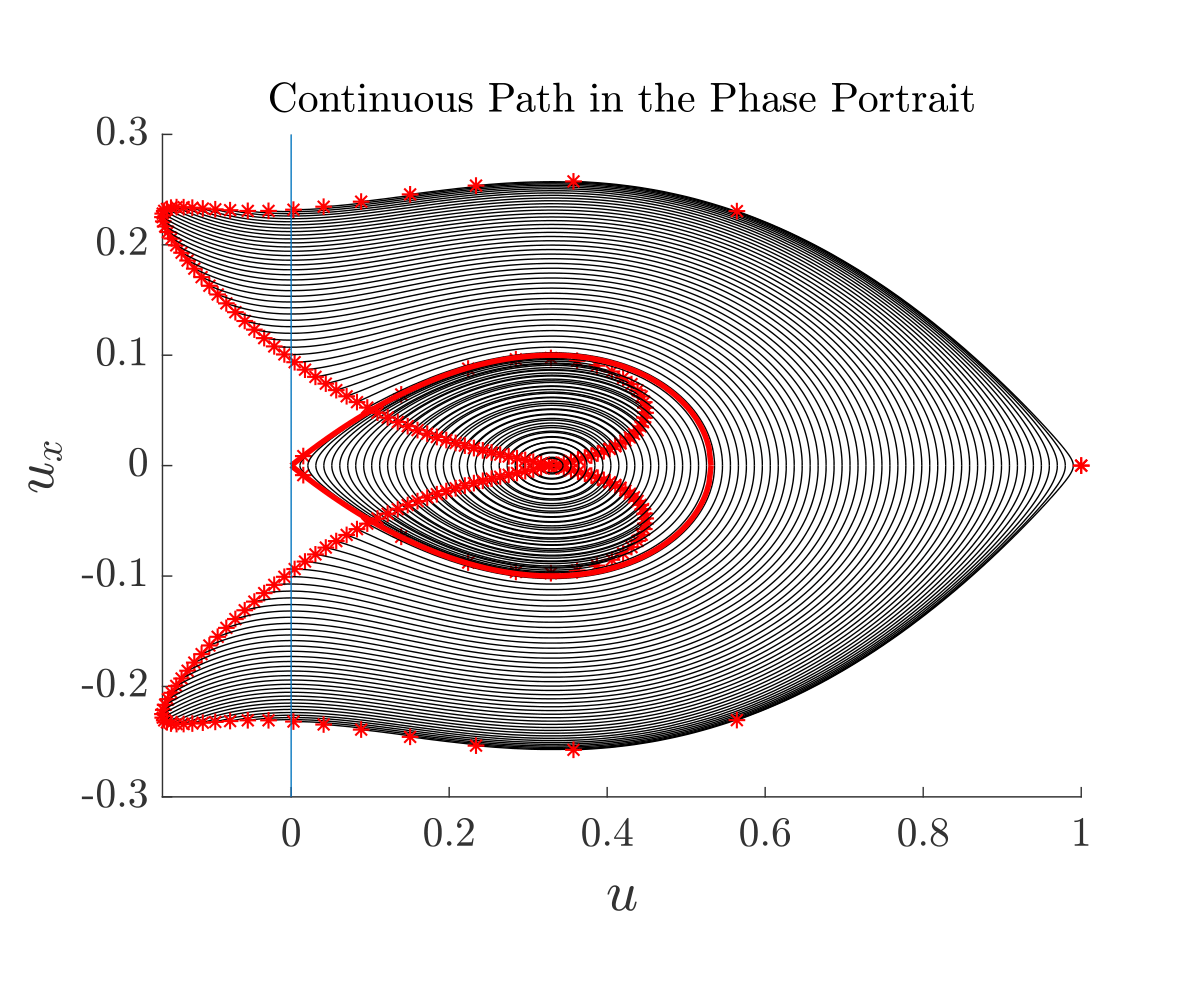• In contrast to the unconstrained case, the presence of constraints induces a minimal controllability time. However, the construction done with the path of steady-states is only a way to control and it requires typically a large time, much larger than the minimal one. In this blog post, we explore different ways to control the system, a minimal controllability time control, a quasistatic control and we explore numerically the effect of the presence of barriers in an optimal control framework.
•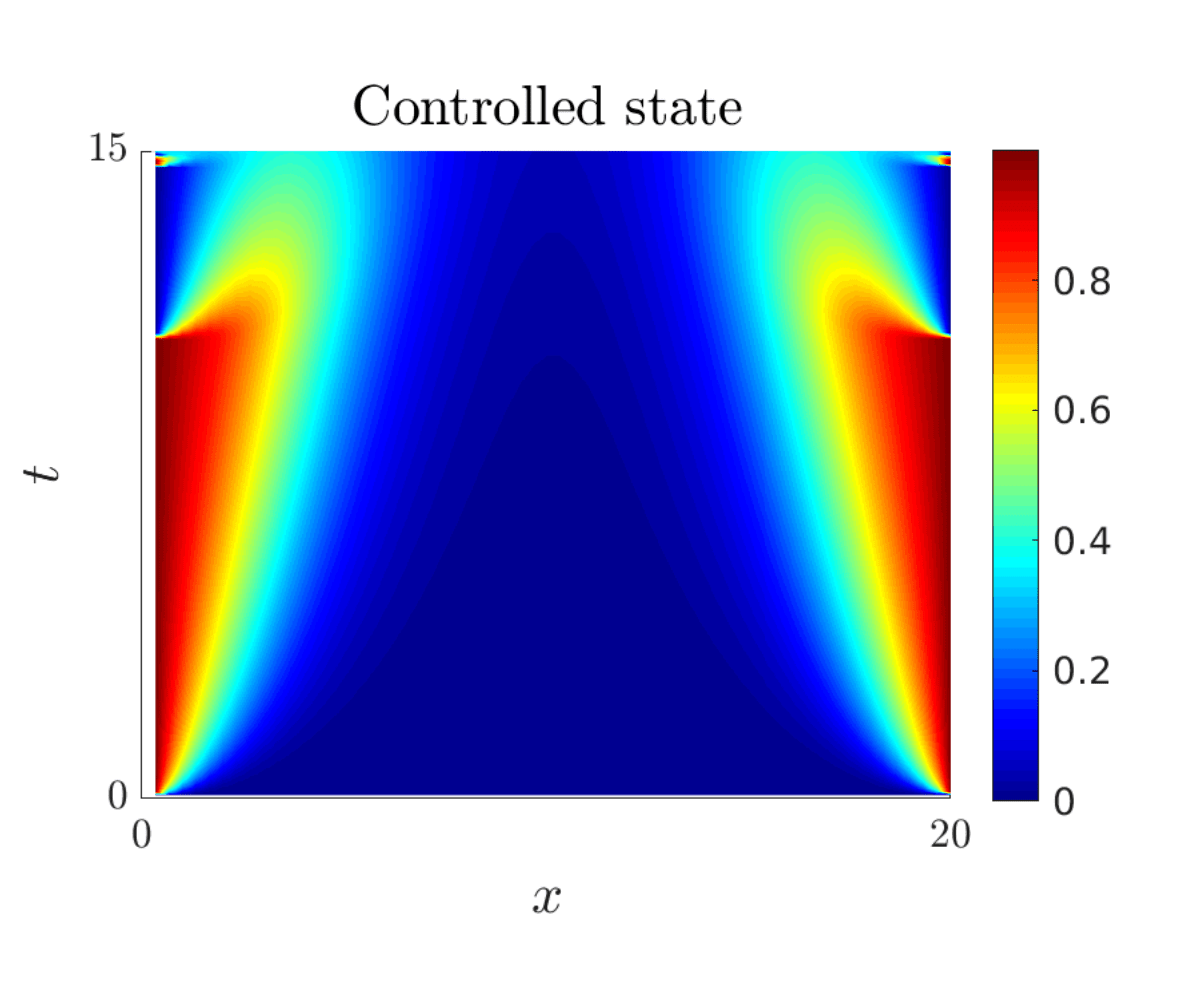• Many models in population dynamics take care about spatial heterogeneity, this can lead for example to new types obstructions. In the blog, we explain the main features and the influence of an heterogeneous drift in a bistable equation called gene-flow. The heterogeneity in the drift comes from an approximation of a system of a system of two equations with an heterogeneous environment.
•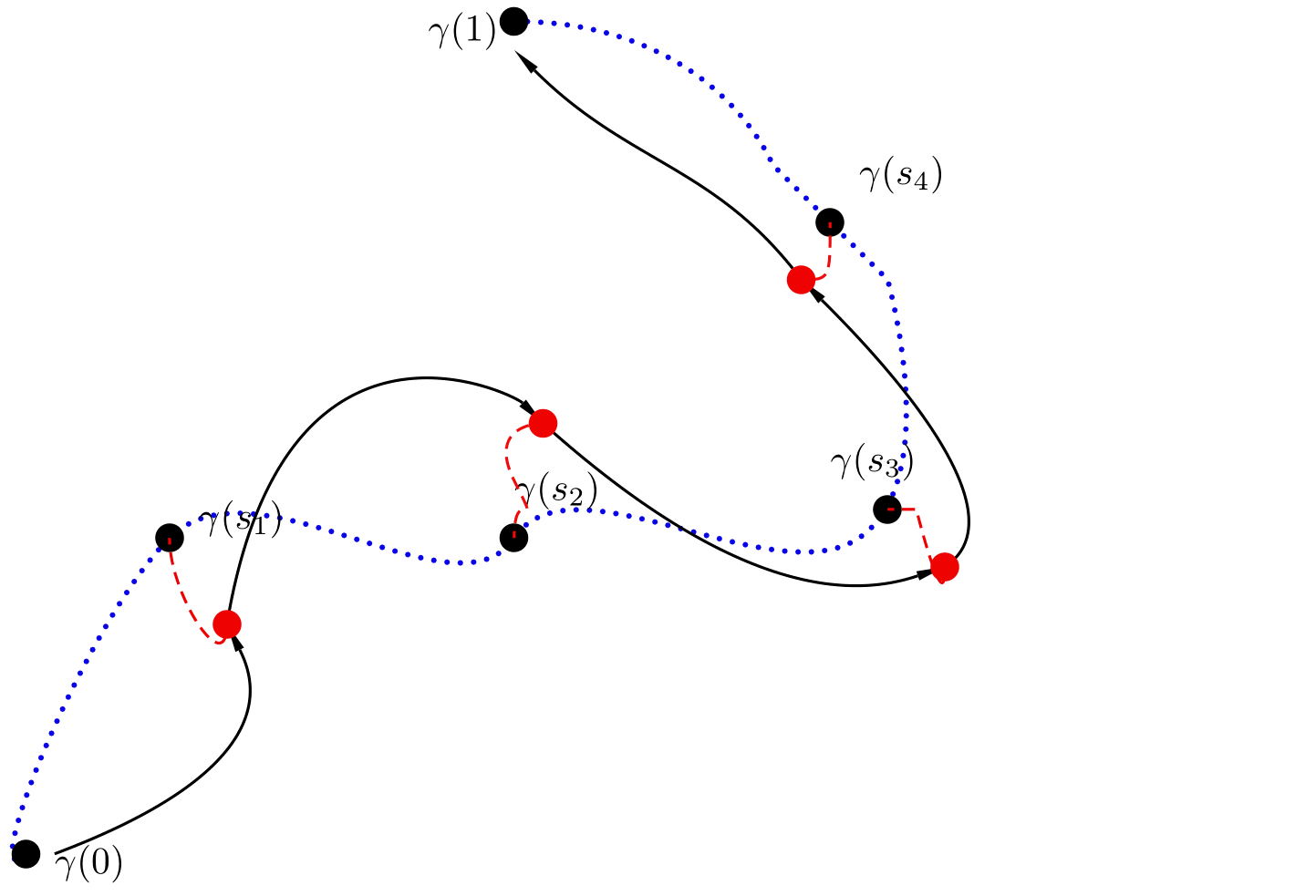A. Kolmogorov, Étude de l’équation de la diffusion avec croissance de la quantité de matière et son application à un problème biologique, Bull. Univ. Moskow, Ser. Internat., Sec. A 1 (1937) 1–25.

 J. Evans, Nerve axon equations 4: the stable and unstable impulse, Indiana Univ. Math. J. 24 (12) (1975) 1169–1190.

 B. Perthame, Parabolic equations in biology : growth, reaction, movement and diffusion, Lecture notes on mathematical modelling in the life sciences, 2015.

 Travelling waves for games in economics and biology, Nonlinear Analysis: Theory, Methods and Applications 30 (2) (1997) 1235 – 1244, proceedings of the Second World Congress of Nonlinear Analysts.

 V. Hutson, K. Mischaikow, G. T. Vickers, Multiple travelling waves in evolutionary game dynamics, Japan Journal of Industrial and Applied Mathematics 17 (3) (2000) 341.

 A. De Masi, P. Ferrari, J. Lebowitz, Reaction diffusion equations for interacting particle systems, J. Stat. Phys. 44 (3-4) (1986) 589–644.

 K. Prochazka, G. Vogl, Quantifying the driving factors for language shift in a bilingual region 114 (17) (2017) 4365–4369.

 M.H. Protter and H.F. Weinberger, Maximum principles in differential equations, Springer Science & Business Media, 2012.

 G. Lebeau, L. Robbiano, Contrôle exact de léquation de la chaleur, Communications in Partial Differential Equations 20 (1-2) (1995) 335–356.

 E. Fernández-Cara and E. Zuazua, Null and approximate controllability for weakly blowing up semilinear heat equations, Ann. Inst. H. Poincaré Anal. Non Linéaire 17 (2000), no. 5, 583 – 616.

 A.V. Fursikov and O.Y. Imanuvilov, Controllability of evolution equations, no. 34, Seoul National University, 1996.

 D. Ruiz-Balet and E. Zuazua. Control of certain parabolic models from biology and social sciences. Preprint available at https://cmc.deusto.eus/domenec-ruiz-balet/.

 P.L. Lions, On the existence of positive solutions of semilinear elliptic equations, SIAM Rev. 24 (1982), no. 4, 441–467.

 D. Pighin, E. Zuazua, Controllability under positivity constraints of multi-d wave equations, in: Trends in Control Theory and Partial Differential Equations, Springer, 2019, pp. 195–232.

 J.-M. Coron, E. Trélat, Global steady-state controllability of one-dimensional semilinear heat equa- tions, SIAM J. Control. Optim. 43 (2) (2004) 549–569.

 C. Pouchol, E. Trélat, E. Zuazua, Phase portrait control for 1d monostable and bistable reac- 635 tion–diffusion equations, Nonlinearity 32 (3) (2019) 884–909.

 D. Ruiz-Balet and E. Zuazua. Controllability under constraints for reaction-diffusionequations: The multi-dimensional case (2019). Preprint available athttps://cmc.deusto.eus/domenec-ruiz-balet/.

 I. Mazari, D. Ruiz-Balet, and E. Zuazua, Constrained control of bistable reaction-diffusion equations: Gene-flow and spatially heterogeneous models, preprint: https://hal.archives-ouvertes.fr/hal-02373668/document (2019).